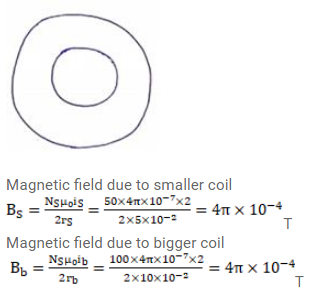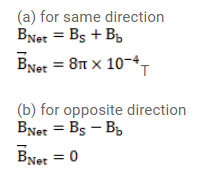# Two circular coils of radii 5.0cm and 10cm carry equal currents of 2.0A.

Question:

Two circular coils of radii $5.0 \mathrm{~cm}$ and $10 \mathrm{~cm}$ carry equal currents of $2.0 \mathrm{~A}$. The coils have 50 and 100 turns respectively and are placed in such a way that their planes as well as the centers coincide. Find the magnitude of the magnetic field B at the common center of the coils if the currents in the coils are

(a) in the same sense.

(b) in the opposite sense.

Solution: